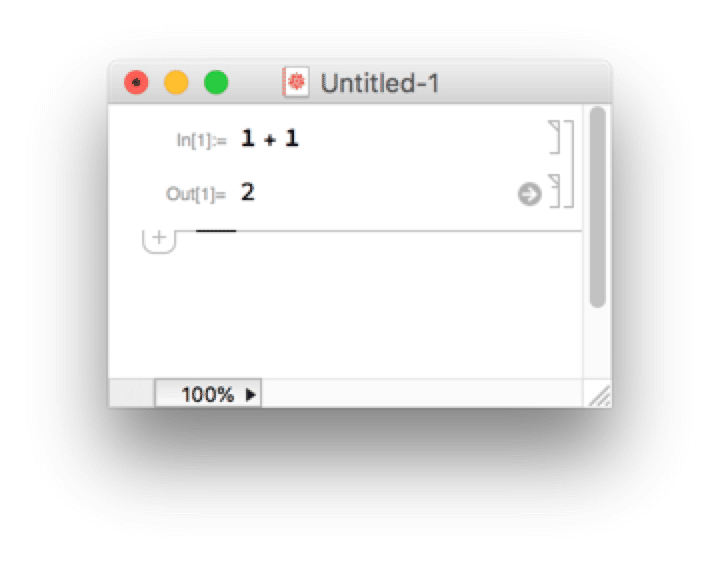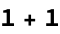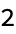Introduction
IntroductionEx (the Wolfram Language for Students - Personal Use Only : www.wolfram.com)

What is Mathematica?

Mathematica is a powerful symbolic computation program running the Wolfram Language. It was created by Stephen Wolfram and is used widely for mathematical, scientific, and engineering applications.

The notebook interface

Very similar to Jupyter notebooks

Enter code/expressions in a cell

Shift-return (or enter on the numeric keypad) evaluates the expression

The output is shown below the input cell